# Calculator for viewing angles

#### Rory

##### Senior Member
Please find attached an excel spreadsheet containing a calculator which works out the viewing (elevation) angles for an observer of a given height on both a flat and sphere earth, from distances and elevations.

This is what it looks like:For the flat earth, it's simple trig - tan(x)=(target height-observer height)/distance - while for the sphere earth, it works by calculating a series of isosceles triangles: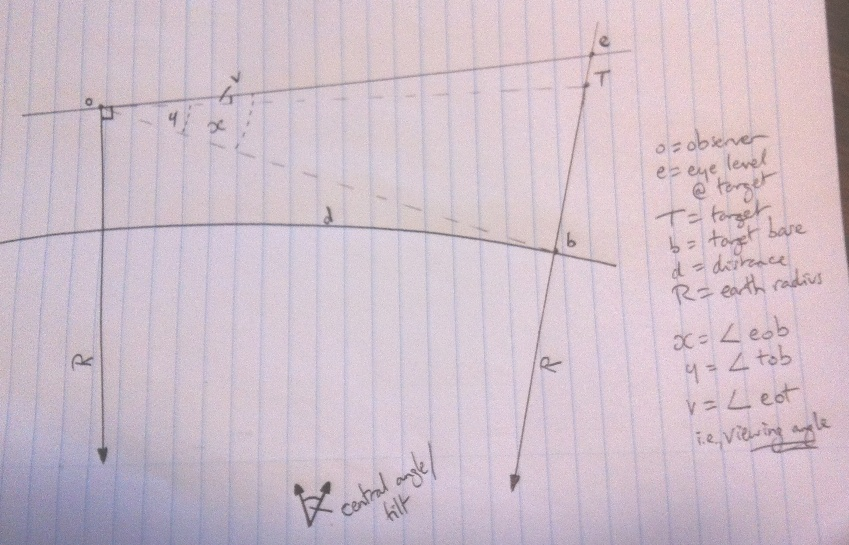I had done this is in a more straightforward way in the past - but like my obstruction calculator, I found that there was an issue in what happens when targets are above or below eye level (i.e., positive and negative angles).

Now it calculates using angular size, which gets around this problem. Basically: angular size of target - angular size of target base to eye level = viewing/elevation angle.

I've checked it against three other methods of calculating the viewing angle, and they all come to the same result (within ~5/1000th of a degree).

This can then be used to check apparent height order in photographs, and even to predict at what approximate pixel height a landmark should appear.

Here is where it predicts the mountains listed above should appear on a sphere earth: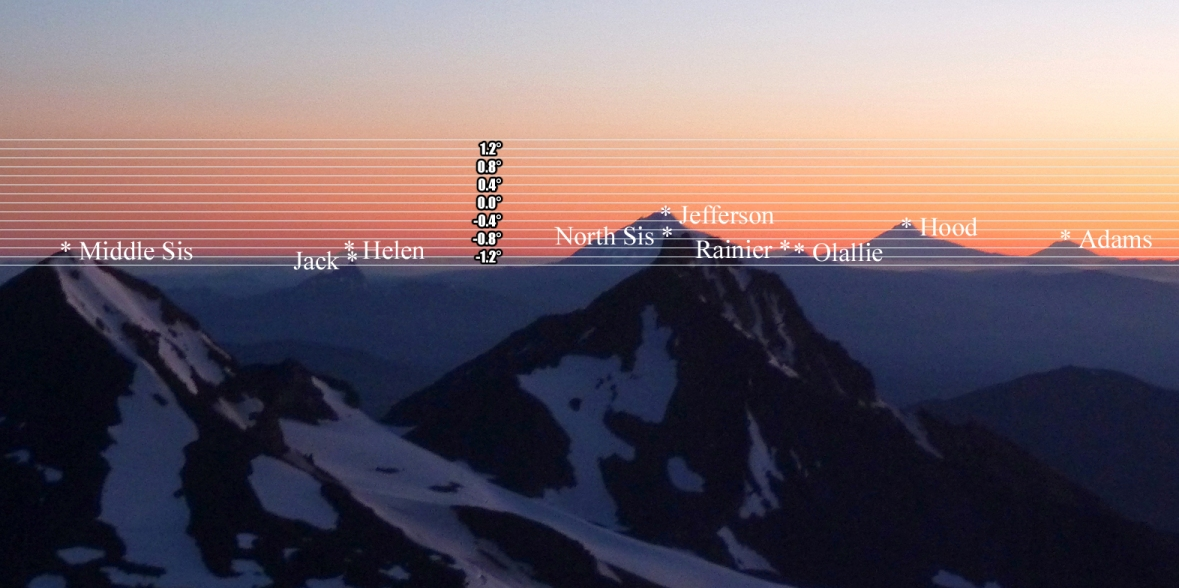And here's where it predicts they should appear on a flat earth: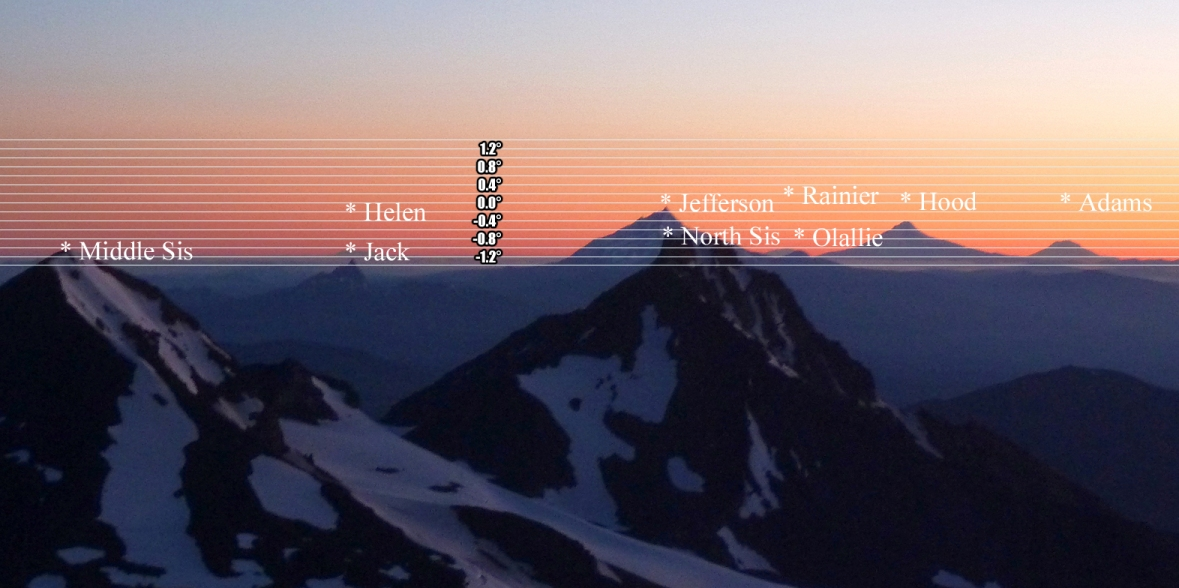I've also tested it over short range, using a photograph of 5th Avenue in New York (more discussion of this here):This time, because of the smaller distances, both models return the same results:The predicted pixel heights for this photo were incredibly accurate, given the rough and ready nature of the method: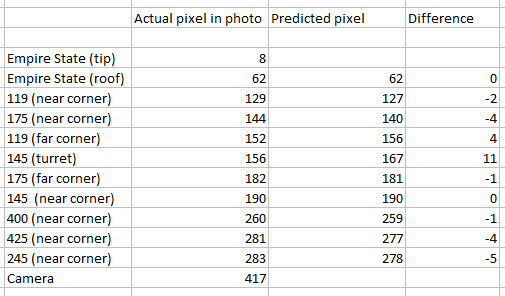This is based on calculating the number of pixels per degree by choosing the highest and lowest landmarks - in this case, the lowest would be the camera, discerned by parallel lines - and then multiplying the generated angles by that number.

Greater accuracy may be obtained by using different landmarks for this figure, or by factoring in the slight incline of 5th Avenue (it's about 20 feet higher near the Empire State than at 17th Street, where the camera was) - but, given that most of them are within 4 pixels, I think it's a pretty satisfying result.#### Attachments

• 37.5 KB Views: 128
Last edited:

#### Rory

##### Senior Member
While for the sphere earth, it works by calculating a series of isosceles triangles:If anyone's curious what I mean by this:
• We know R (earth's radius, derived here from latitude), o (observer height), and T (target height)
• Central angle can be calculated by d (distance) over R
• The line ob can then be calculated using Ro, R, and the central angle
• In the same way, oT is calculated (this time RT instead of R)
• Now knowing the three side oT, ob, and bT, y can be calculated (target angular size)
• The lines oe is calculated by Ro, the central angle, and 90 degrees
• Re is calculated likewise, then R subtracted to give be
• Now I can find the angle eob (x)
• x-y gives the viewing angle, v
There may be a quicker and/or better way to arrive at v, but this is the best that my knowledge could do.

Cheers.#### Mendel

##### Member
@Rory your "viewing angle" is called "angle of elevation" by everyone else, or "angle of depression" when it is downward as in your sketch. If you add 90°, it's a "zenith angle", since it's then relative to the straight-up direction.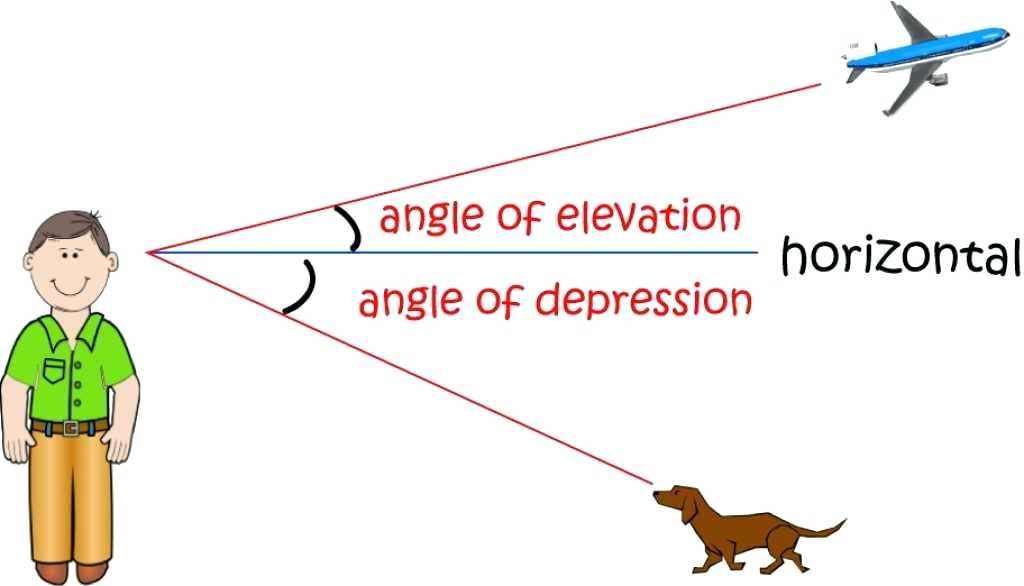#### Rory

##### Senior Member
@Rory your "viewing angle" is called "angle of elevation" by everyone else, or "angle of depression" when it is downward.
Not to worry; they'll catch on."Viewing angle" is good because it's more of a layman's term for a layman's subject, and it encompasses both "elevation" and "depression", rather than needing two terms depending on a plus or minus angle.

#### Mendel

##### Member
@Rory I'm concerned that people could mistake your "viewing angle" for the angular size. It also has the drawback of misleading people trying to look it up on search engines.

"Angle of elevation" is the general term, and a negative elevation angle is understood to be a depression.

#### deirdre

##### Moderator
Staff member
I'm concerned that people could mistake your "viewing angle" for the angular size.
line-of-sight angle might be better for laymen. (but now that search term should hit this thread as i just typed it).

"Predicted" is what bothers me. Psychics predict. My math teacher never said "the predicted answer is 4", he (or she) would say "the answer is 4".

#### Mick West

Staff member
"Predicted" is what bothers me. Psychics predict. My math teacher never said "the predicted answer is 4", he (or she) would say "the answer is 4".
It's common in science when discussing models to talk about the predictions they make
https://scienceornot.net/2012/01/17/scientific-models-are-tested-by-making-predictions-and-checking-them-against-real-world-data/
Here we've got two competing models, Flat and Globe. So to test them we see what they predict, and we test the predictions.

Often this comes with progressive refinements of the model, as the test reveals areas of inaccuracy. Like you'd go from a spere to an oblate spheroid, and from ignoring the atmosphere to including the atmosphere.

#### Mendel

##### Member
line-of-sight angle might be better for laymen. (but now that search term should hit this thread as i just typed it).

"Predicted" is what bothers me. Psychics predict. My math teacher never said "the predicted answer is 4", he (or she) would say "the answer is 4".
"line-of-sight angle" is correct terminology, so it works for non-laymen as well.Mathematics has no predictions, because it doesn't use observations to check its results. You prove that 2+2=4, given the axioms and definitions, and that's that. But you can't ever prove anything in science, we just accept the theories that give us the best descriptions of reality. And the criterium for "best description" is "most accurate prediction of future observations", which is pretty important if you're an engineer and want to predict what happens when you turn your newly designed device on, or a civil engineer predicting what your bridge will do when a truck passes it in a strong wind.

Or, to be historical, astronomical predictions are very important if you're determining the position of your ship by celestial navigation. In Kepler's time, astronomical tables would be accurate for about a year in advance, but his laws of planetary motion enabled him to be accurate for more than a decade into the future: his tables were accurate to 20 arc seconds, as opposed to 5 degrees accuracy for earlier tables, which enabled easier navigation and showed the superiority of using elliptical heliocentric orbits to describe planetary motion.

Another difference is that scientific predictions and measurements come with margins of error, while mathematical results don't. (Mathematics is basically a branch of philosophy.)

#### deirdre

##### Moderator
Staff member
Well, as a layman, if i hear the word "calculator" i expect the answer given to be the answer.

#### Rory

##### Senior Member
So I just did a check on how the super simple way of calculating these viewing angles compares to the long and accurate way, and what I found is...the maximum variation for all the above examples was 0.001° (and more than half of them were accurate to within 0.0002°).

What do I mean by the simple way? Well, the flat angle is calculated by tan(x)=elevation/distance, and the sphere angle can be calculated by tan(x)=(elevation-refracted drop)/distance.

Note: elevation = elevation above or below the observer, not sea/ground level.

It's good to have done it the complex way - and it's equally good to see that the simple way works just as well, for all practical purposes.
Well, as a layman, if i hear the word "calculator" i expect the answer given to be the answer.
It is the answer. To the question "what is the predicted amount"?I think the thing here is, we know it won't be exactly right. There are too many variables, such as changes in atomspheric conditions, exactly accurate measurements, the camera, the image. But if we say, well, it predicts these landmarks will be at pixels 12, 160, and 310, and they turn out to be at 11, 162, and 306, we can be pretty happy with the results.

I guess if it was a purely mathematical scenario, we would expect an "answer" rather than a "prediction" - if I have 10 apples and I take 8 away, etc - but I don't think we can say that here. For example, the predicted height order may not be perfectly right - especially if we have four landmarks separated by just a pixel or two, as we do with the South Sister photo.

#### deirdre

##### Moderator
Staff member
But if we say, well, it predicts these landmarks will be at pixels 12, 160, and 310, and they turn out to be at 11, 162, and 306, we can be pretty happy with the results.
OH! so its a calculator for predicting line-of-sight angles in PHOTOGRAPHS. ok i get it now.

I was thinking you should add some text to your calculator. When i looked at it i noticed i could download and save it. SO for people who share it and dont read this thread, some caveats written on it would help.
Like i tried to put a figure in above your "Mount Jeffersin" and nothing happened. SO finally i deleted the MT JEfferson numbers (over-wrote) them... but maybe tell people they have to do that or add the formulas tot he empty space above Jefferson so we can add or own stuff without messing with your lines. (which is weird, i thought spreadsheets attached the formulas to whole columns)

#### Rory

##### Senior Member
Good idea: I'll do that. Ideally it would be on a webpage somewhere but I've got no idea how to do that.

At the basic level it's for predicting the order things will appear in both photos or reality - i.e., what will seem higher than what - but the pixel position thing is a nice bonus.

#### Mendel

##### Member
@deirdre you can measure these angles in reality with a theodolite (app) or a sextant. Because they are in reality, they are also in photographs of reality.

@Rory I like to use "height" when referring to a vertical distance (as opposed to a vertical angle) to avoid confusion, as in "height above the observer" of "height above sea level". Elevation is sometimes used for "height above sea level", but we already need the word to refer to the angle.